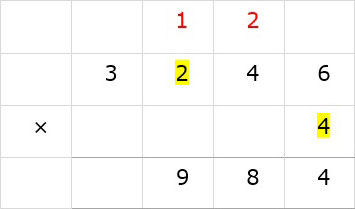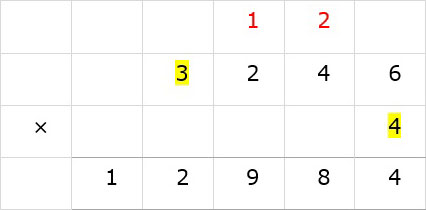# Multiplication of a single digit number with large numbers

Multiply 3246 × 4

### Solution

Step 1:

First we multiply 4 with 6 of 3246, 4 × 6 = 24. We bring down 4 and carry 2 to tens column.Step 2:

Next we multiply 4 with 4 of 3246, 4 × 4 = 16. 16 + 2 = 18. We bring down 8 and carry 1 to hundreds column.Step 3:

Next we multiply 4 with 2 of 3246, 4 × 2 = 8. 8 + 1 = 9. We bring down 9.Step 4:

Next we multiply 4 with 3 of 3246, 4 × 3 = 12. We bring down 12 as shown to get the final answer as 12984.